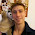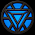### Deriving the Time-Dependent Schrödinger Equation $\hat{\mathcal{H}}\Psi=E\Psi$

Good morning fellow mathematicians! Another week, another post. Just like the title says, we are going to "derive" the Time-Dependent Schrödinger Equation today in a very intuitive way, using well-known concepts that you might encounter in classical mechanics. At first I would like you to remember the Maxwell Equations in free space in their differential form:

$$\vec{\nabla} \times \vec{E}=-\partial_t \vec{B} \qquad\qquad \vec{\nabla} \times \vec{B}=\frac{1}{c^{2}}\partial_t \vec{E}\\ \vec{\nabla}\circ\vec{E}=0 \qquad\qquad \vec{\nabla}\circ\vec{B}=0$$

Now, what would happen if we would apply a curl to $\vec{\nabla} \times \vec{E}$ ? On the one hand we could make use of the other Maxwell Equations and by interchanging the differentials to get:

\begin{align*} \vec{\nabla}\times(\vec{\nabla} \times \vec{E})&=\vec{\nabla}\times(-\partial_t \vec{B})\\ &=-\partial_t (\vec{\nabla}\times\vec{B})\\ &=-\partial_t (\frac{1}{c^{2}}\partial_t \vec{E})\\ &=-\frac{1}{c^{2}}\partial_t^{2} \vec{E} \end{align*}

On the other hand, we can make use of a well-known vector identity and another one of the Maxwell Equations to arrive at the conclusion, that:

\begin{align*} \vec{\nabla}\times(\vec{\nabla} \times \vec{E})&=\vec{\nabla}(\vec{\nabla}\circ\vec{E})-\vec{\nabla}^2\vec{E}\\ &=-\vec{\nabla}^2\vec{E} \end{align*}

Now here comes the fun part. By setting both expressions equal, we can actually get our hands on the 3-dimensional wave equation, a partial differential equation which plays a big role in classical mechanics:

$$-\vec{\nabla}^2\vec{E}=-\frac{1}{c^{2}}\partial_t^{2} \vec{E}\\ \iff [\vec{\nabla}^2-\frac{1}{c^{2}}\partial_t^{2}] \vec{E}=0\\$$

We can rewrite this last differential operator as just $\Box$ which is widely known as the d'Alembertian, to get $\Box\vec{E}=0$. This is looking quite simple now, doesn't it? As a matter of fact, there are many solutions, so-called wave functions, that satisfy the given partial differential equation. One of those solutions are monochromatic plane waves, which come in the form of

$$\Psi(x,t)=\Psi_{0}e^{i(kx-\omega t)}\text{ ,}$$

where $\Psi_{0}$ is the amplitude, $k$ (strictly speaking it's $\vec{k}$, but let us consider the wave function in one dimension for now) is the wavenumber and $\omega$ is the frequency of the system.

Let us now go quantum! By identifying $\Psi$ as a particle wave, we can actually make use of de Broglie's hypothesis $p=\hbar k$ and the Planck-Einstein relation $E=\hbar\omega$, to substitute the values for $\omega$ and $k$ respectively:

\begin{align*} \Psi(x,t)&=\Psi_{0}e^{i(kx-\omega t)}\\ &=\Psi_{0}e^{i(\frac{p}{\hbar}x-\frac{E}{\hbar}t)}\\ &=\Psi_{0}e^{\frac{i}{\hbar}(px-Et)} \end{align*}

A lot of the work has already been done! All that is left to do, is to consider the conservation of energy in physical systems, namely $E=T+V$, where $E$ is the whole energy of the system, with $T$ being the kinetic energy and $V$ being the potential our particle is in. As long as we are not dealing with a trivial wave function, meaning $\Psi(x,t)=0$, we can safely multiply both sides of our conservation law by it, giving us

$$E\Psi(x,t)=T\Psi(x,t)+V(x)\Psi(x,t) \text{ ,}$$

where we can express the kinetic energy as $T=\frac{p^2}{2m}$, with $p$ being the momentum and $m$ the mass of the particle.

Instead of simply substituting our $\Psi$ in, we could also make use of the fact, that it is a function in two dimensions. This basically allows us to partially differentiate our function in both variables, providing us with

$$\partial_{t}\Psi=-\frac{i}{\hbar}E\Psi\\ \iff i\hbar\partial_{t}\Psi=E\Psi$$

and

$$\partial_{x}^{2}\Psi=-\frac{p^2}{\hbar^2}\Psi\\ \iff p^2\Psi=-\hbar^2\partial_{x}^{2}\Psi \text{ .}$$

Once again, I'm leaving it as an exercise for the reader to verify the correctness of those differentials. Now we can safely substitute those newly gathered values into our modified conservation of energy law to finally arrive at the conclusion:

$$i\hbar\partial_{t}\Psi=-\frac{\hbar^2}{2m}\partial_{x}^{2}\Psi+V(x)\Psi$$

And here it is, our finished, fresh out of the oven Schrödinger's Equation in one dimension! If you want to go 3-D, feel free to transform our differential operator $\partial_{x}^2$ into $\vec{\nabla}^2$ to get the full quantum experience.

And this is it for today! I hope you did enjoy the read. If you did, feel free subscribing to my blog and to leave some constructive criticism down below. I thank you guys for your attention and up until the next post,

--have a flammable day $\int d \tau$1.And once again a very nice post! I did not expect anything less from you! :D

2.And I am the first again XD

1.Hehe :D Thank you for the nice words! :3

3.1.4.You could have just started from stating its a wave, then you have wave equation for free. In fact, starting from Maxwell's equation complicates things. First, the electric field is not a complex number. Your $\Psi$ is a complex number you have to take the real part of it. To be more precise, you have to consider polarization as well. Second, the Maxwell equation you used does not contain particles but the Schroedinger's equation you derived does, and it is the opposite. Third, Maxwell's equation is intrinsically relativistic. You should have sticked with the D'Alembertian and come up with the Kleine-Gorden Equation instead of using $T=\frac{p^2}{2m}$ and derived the Schroedinger's equation.

1.The electric field is in general a complex function - that is to say that finding solutions to the wave equation which are complex are in fact still valid solutions, and indeed some solutions may only be found this way. The real part must be taken to obtain the physical field though - about this you are correct.

The wavefunction solutions of the Schrödinger eqn are very often complex, but taking the real part won't net you anything physical. You need to take the integral of the wave function multiplied by its complex conjugate (aka the abs value squared) if you want to arrive at the probability of finding a particle within the range of your integral.

Plarization is accounted for, the E field is a vector field. It is already baked in :)

5.I wish i could understand this shit nibba.

1.6.1.2.7.papa you are the beast

1.8.How did you do Latex on blogger? Did you pay a fee?

1.No, I was just using a Mathjax html script.

9.I would just start with the wave equation; starting from electromagnetism might encourage new learners to think the Schrodinger equation is somehow necessarily linked to it.